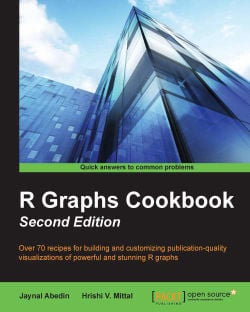R Graphs Cookbook (Second Edition)4.3 (3 reviews total)
By Jaynal Abedin , Hrishi V. Mittal
• \$5/mo for 5 months Subscribe Access now
• \$32.99 eBook

• Constantly updated with 100+ new titles each month
• Breadth and depth in over 1,000+ technologies

Data visualization is one of the most important tasks in the data science track. Through effective visualization, we can easily uncover underlying patterns among variables without doing any sophisticated statistical analysis.

Starting with a high-level overview of the R graphics system and then moving through this practical cookbook, you will leverage powerful default R graphics and utilize advanced graphics systems such as lattice and ggplot2, the grammar of graphics. Through inspecting large datasets using tableplot and stunning three-dimensional visualizations, you will know how to produce, customize, and publish advanced visualizations using this popular, and powerful, framework.

Publication date:
October 2014
Publisher
Packt
Pages
368
ISBN
9781783988785

Chapter 1. R Graphics

R provides a number of well-known facilities that produce a variety of graphs to meaningfully visualize data. It has low-level facilities where we deal with basic shapes to draw graphs and high-level facilities. There are functions available here to produce quality graphs; these functionalities are usually developed using certain combinations of basic shapes. Using R, we can produce traditional plots, the trellis plot, and very high-level graphs inspired by the Grammar of Graphics implemented in the ggplot2 package. The default graphics package is useful for traditional plots, lattice provides facilities to produce trellis graphs, and the ggplot2 package is the most powerful high-level graphical tool in R. Other than these, there are low-level facilities that draw basic shapes, and arranging the shapes in their relative position is an important step in order to create meaningful data visualization. In this chapter, we will introduce both low-level graphics (also known as base graphics) and high-level graphics using different packages. Particularly, the content of this chapter will be as follows:

• Base graphics using the default package

• Trellis graphs using lattice

• Graphs inspired by Grammar of Graphics

Base graphics using the default package

It is well known that R has very powerful data visualization capabilities. The primary reason behind the powerful graphical utility of R is the low-level graphical environment. The grid graphic system of R makes data visualization much more flexible and intuitive. With the help of the grid package, we can draw very basic shapes that can be arranged to produce interesting data visualizations. There are functions in the grid graphics system that draw very basic shapes of a high-level data visualization, including lines, rectangles, circles, and texts along with some other functions that specify where to put which part of the visualization. Through the use of the basic function, we can easily produce components of high-level graphs, such as a rectangle, rounded rectangle, circle, line, and arrow. We will now see how we can produce these basic shapes. In a single visualization, we will show you all the output from the following code snippet:

# Calling grid library
library(grid)

# Creating a rectangle
grid.rect(height=0.25,width=0.25)

# A rounded rectangle
grid.roundrect(height=0.2,width=0.2)

# A circle
grid.circle(r=0.1)

# Inserting text within the shape
grid.text("R Graphics")
# Drawing a polygon
grid.polygon()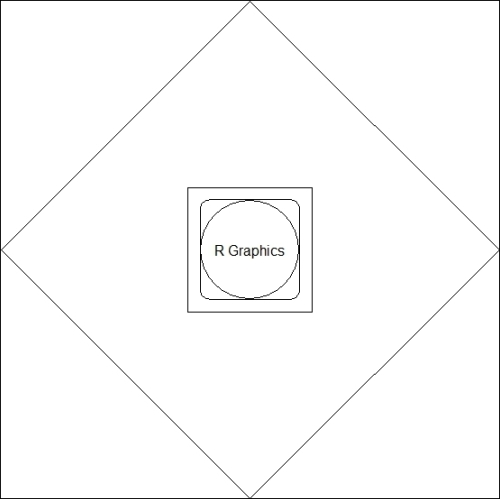Basic shapes using the grid package

For any high-level visualization, we can use the basic shapes and arrange them as required. Now, we will list some of the functions for high-level data visualization where the basic shapes have been used:

• plot: This is a generic function that is used to plot any kind of objects. Most commonly, we use this function for x-y plotting

• barplot: This function is used to produce a horizontal or vertical bar plot

• boxplot: This is used to produce a box-whisker plot

• pie: This is used to produce a pie chart

• hist: This is used to produce a histogram

• dotchart: This is used to produce cleveland dot plots

• image, heatmap, contour, and persp: These functions are used to generate image-like plots

• qqnorm, qqline, and qqplot: These functions are used to produce plots in order to compare distributions

We will provide specific recipes for each of these functions in the subsequent chapters.

Trellis graphs using lattice

Though grid graphics have much more flexibility than trellis graphs, it is a bit difficult to use them from the point of view of general users. The lattice package enhances the data visualization capability of R through relatively easy code in order to produce much more complex graphs. This allows the user to produce multivariate visualization. The lattice package could be considered as a high-level data visualization tool that is able to produce structured graphics with the flexibility to adjust the graphs as required.

The traditional R graphics system has much more flexibility to produce any kind of data visualization with control over each and every component. However, it is still a difficult task for an inexperienced R programmer to produce efficient graphs. In other words, we can say that the traditional graphic system of R is not so user friendly. It would be good if the user could have complete high-level graphics with the use of minimal written code. To address this shortcoming, Trellis graphics have been implemented in S. The inspired lattice add-on package is the add-on package that provides similar capabilities for R users. One of the important features of the lattice graphics system is the formula interface. During data visualization, we can intuitively use the formula interface to produce conditional plots, which is difficult in a traditional graphics system.

For example, say we have a dataset with two variables, an incubation period, and the exposure category of a certain disease. This dataset contains one numeric variable, the incubation period itself, and another discrete variable with four possible values: 1, 2, 3, or 4. We want to produce a histogram for each exposure category. The following code snippet shows you the traditional code:

# data generation

# Set the seed to make the example reproducible
set.seed(1234)
incubation_period <- c(rnorm(100,mean=10),rnorm(100,mean=15),rnorm(100,mean=5),rnorm(100,mean=20))
exposure_cat <- sort(rep(c(1:4),100))
dis_dat<-data.frame(incubation_period,exposure_cat)

# Producing histogram for each of the exposure category 1, 2, 3, and 4
# using traditional visualization code. The code below for
# panel histogram for different values of the variable
# exposure_cat. This code will produce a 2 x 2 matrix where
# we will have four different histograms.
op<-par(mfrow=c(2,2))
hist(dis_dat\$incubation_period[dis_dat\$exposure_cat==1])
hist(dis_dat\$incubation_period[dis_dat\$exposure_cat==2])
hist(dis_dat\$incubation_period[dis_dat\$exposure_cat==3])
hist(dis_dat\$incubation_period[dis_dat\$exposure_cat==4])
par(op)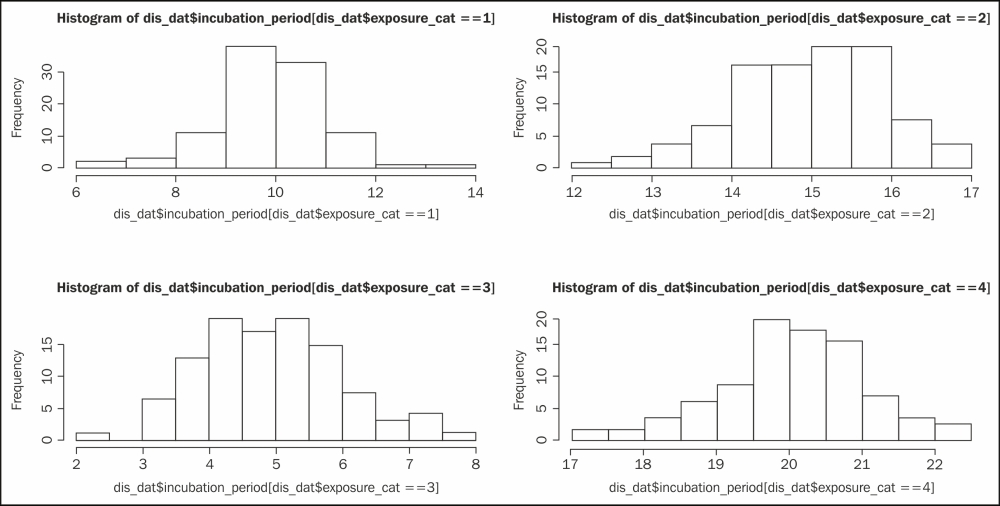The following code snippet shows you the lattice implementation for the same histogram:

library(lattice)
histogram(~incubation_period | factor(exposure_cat), data=dis_dat)

In this lattice version of the code, it is much more intuitive to write the entire code to produce a histogram using the formula interface. The code that follows the ~ symbol contains the name of the variable that we are interested in to produce the histogram, and then we specify the grouping variable. The ~ symbol acts like the of preposition, for example, the histogram of the incubation period. The vertical bar is used to represent the panel variable over which we are going to repeat the histogram. Notice that we have used the factor command here to specify the grouping variable. If we do not specify the factor, then we will not be able to distinguish which plot corresponds to which category. The factor()command creates text labels. If the variable was left as a numeric value, it would show low to high values as though it were a continuous scale rather than discrete categories, as shown in the following figure: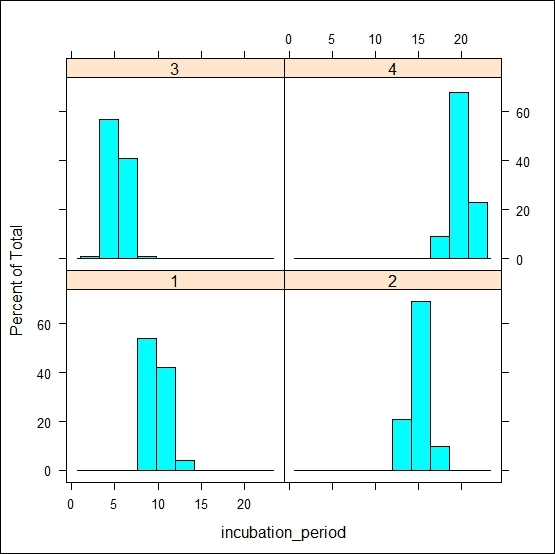Now, if we change the code's formula part and use a plot generic function instead of the histogram, then the visualization will be changed as follows:

plot(incubation_period ~ factor(exposure_cat), data=dis_dat)

Tip

You can download the example code files for all Packt books you have purchased from your account at http://www.packtpub.com. If you purchased this book elsewhere, you can visit http://www.packtpub.com/support and register to have the files e-mailed directly to you.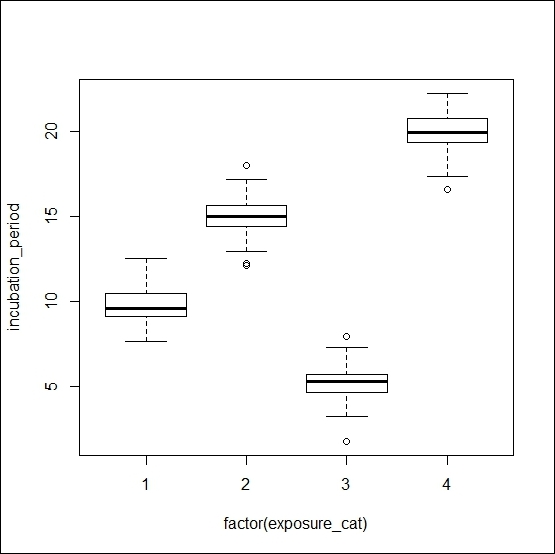If we change the code further and just omit the factor function, then the same visualization will be turned into a scatter plot as follows:

plot(incubation_period ~ exposure_cat, data=dis_dat)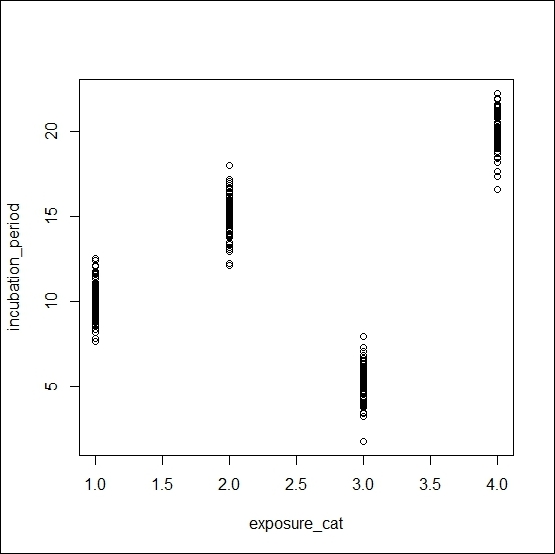The plot()function is a generic function. If we put two numeric variables inside this function, it produces a scatter. On the other hand, if we use one numeric variable and another factor variable, then it produces a boxplot of the numeric variable for each unique value of the factor variable.

Graphs inspired by Grammar of Graphics

The ggplot2 R package is based on The Grammar of Graphics by Leland Wilkinson, Springer). Using this package, we can produce a variety of traditional graphics, and the user can produce their customized graphs as well. The beauty of this package is in its layered graphics facilities; through the use of layered graphics utilities, we can produce almost any kind of data visualization. Recently, ggplot2 has become the most searched keyword in the R community, including the most popular R blog (www.r-bloggers.com). The comprehensive theme system allows the user to produce publication quality graphs with a variety of themes of their choice. If we want to explain this package in a single sentence, then we can say that if whatever we can think about data visualization can be structured in a data frame, the visualization is a matter of few seconds.

In Chapter 12, Data Visualization Using ggplot2, on ggplot2 , we will see different examples and use themes to produce publication quality graphs. However, in this introductory chapter, we will show you one of the important features of the ggplot2 package that produces various types of graphs. The main function is ggplot(), but with the help of a different geom function, we can easily produce different types of graphs, such as the following:

• geom_point(): This will create a scatter plot

• geom_line(): This will create a line chart

• geom_bar(): This will create a bar chart

• geom_boxplot(): This will create a box plot

• geom_text(): This will write certain text inside the plot area

Now, we will see a simple example of the use of different geom functions with the default mtcars dataset in R:

library(ggplot2)
# creating a basic ggplot object
p <- ggplot(data=mtcars)
# Creating scatter plot of mpg and disp variable
p1 <- p+geom_point(aes(x=disp,y=mpg))
# creating line chart from the same ggplot object but different
# geom function
p2 <- p+geom_line(aes(x=disp,y=mpg))
# creating bar chart of mpg variable
p3 <- p+geom_bar(aes(x=mpg))
# creating boxplot of mpg over gear
p4 <- p+geom_boxplot(aes(x=factor(gear),y=mpg))
# writing certain text into the scatter plot
p5 <- p1+geom_text(x=200,y=25,label="Scatter plot")

The visualization of the preceding five plots will look like the following figure: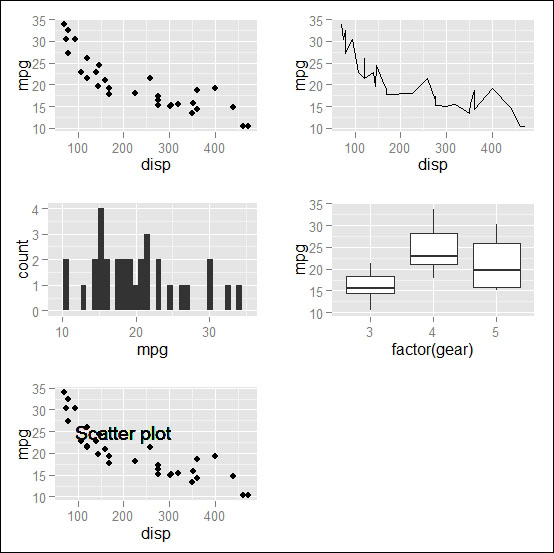• Jaynal Abedin

Jaynal Abedin is currently doing research as a PhD student at Unit for Biomedical Data Analytics (BDA) of INSIGHT at the National University of Ireland Galway. His research work is focused on the sports science and sports medicine area in a targeted project with ORRECO --an Irish startup company that provides evidence-based advice to individual athletes through biomarker and GPS data. Before joining INSIGHT as a PhD student he was leading a team of statisticians at an international public health research organization (icddr,b). His primary role there was to develop internal statistical capabilities for researchers who come from various disciplines. He was involved in designing and delivering statistical training to the researchers. He has a bachelors and masters degree in statistics, and he has written two books in R programming: Data Manipulation with R and R Graphs Cookbook (Second Edition) with Packt. His current research interests are predictive modeling to predict probable injury of an athlete and scoring extremeness of multivariate data to get an early signal of an anomaly. Moreover, he has an excellent reputation as a freelance R programmer and statistician in an online platform such as upwork.

Browse publications by this author
• Hrishi V. Mittal

Hrishi V. Mittal has been working with R for a few years in different capacities. He was introduced to the exciting world of data analysis with R when he was working as a senior air quality scientist at King's College, London, where he used R extensively to analyze large amounts of air pollution and traffic data for London's Mayor's Air Quality Strategy. He has experience in various other programming languages but prefers R for data analysis and visualization. He is also actively involved in various R mailing lists, forums, and the development of some R packages.

Browse publications by this author

Latest Reviews

(3 reviews total)